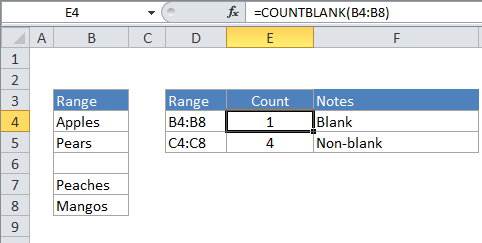## Excel Office

Excel How Tos, Tutorials, Tips & Tricks, Shortcuts

# Count cells that are blank in Excel

This tutorial shows how to Count cells that are blank in Excel using the example below;

## Formula

`=COUNTBLANK(range)`## Explanation

To count the number of cells that are blank, you can use the COUNTBLANK function.

In the example, the active cell contains this formula:

`=COUNTBLANK(B4:B8)`

### How this formula works

COUNTIF counts the number of cells in the range that don’t contain any value and returns this number as the result. Cells that contain text, numbers, dates, errors, etc. are not counted.

Also See:   Count cells equal to either x or y in Excel

You can easily count cells that are not blank using COUNTA function with the same range like so:

`=COUNTA(B4:B8)`

1.